# Physics - Online Test

Q1. The speed of light in an isotropic medium depends on,
Explaination / Solution:

c = vλ, v is independent of medium

Q2. A plane glass is placed over a various coloured letters (violet, green, yellow, red) The letter which appears to be raised more is,
Explaination / Solution:

The refractive index (n) of the glass is different for different colours. For violet n is high. ∴ Violet travel with low speed, so deviation is high.

Q3. Two identical point charges of magnitude –q are fixed as shown in the figure below. A third charge +q is placed midway between the two charges at the point P. Suppose this charge +q is displaced a small distance from the point P in the directions indicated by the arrows, in which direction(s) will +q be stable with respect to the displacement?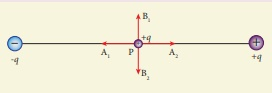Explaination / Solution:

Hint: Since V=0 along equatorial line
Q4. The wavelength λe of an electron and λp of a photon of same energy E are related by
Explaination / Solution:

de Broglie wavelength for an electron

λe= h / √(2mE) ;

λe 1/√E ;

λe2 1/E  ------------- (1)

For photon

λp= hc / E ;

λp 1/E  ------------- (2)

From equation (1) and (2), we have So,

λp λe2

Q5. The following graph shows current versus voltage values of some unknown conductor. What is the resistance of this conductor?Explaination / Solution:Q6. Suppose an alpha particle accelerated by a potential of V volt is allowed to collide with a nucleus whose atomic number is Z, then the distance of closest approach of alpha particle to the nucleus is
Explaination / Solution: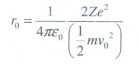When alpha particle is accelerated at the potential V;

K.E = 2eV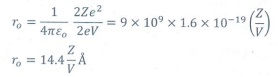Q7. The magnetic field at the center O of the following current loop isExplaination / Solution:Q8. The barrier potential of a silicon diode is approximately,
Explaination / Solution:
No Explaination.

Q9. An electron moves on a straight line path XY as shown in the figure. The coil abcd is adjacent to the path of the electron. What will be the direction of current, if any, induced in the coil?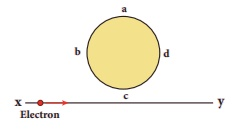Explaination / Solution: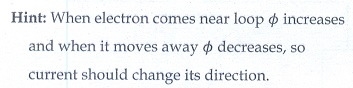Q10. The particle size of ZnO material is 30 nm. Based on the dimension it is classified as
Explaination / Solution:
No Explaination.